# 2-3 Trees | (Search and Insert)

2-3 tree is a tree data structure in which every internal node (non-leaf node) has either one data element and two children or two data elements and three children. If a node contains one data element leftVal, it has two subtrees (children) namely left and middle. Whereas if a node contains two data elements leftVal and rightVal, it has three subtrees namely left, middle and right.

The main advantage with 2-3 trees is that it is balanced in nature as opposed to a binary search tree whose height in the worst case can be O(n). Due to this, the worst case time-complexity of operations such as search, insertion and deletion isas the height of a 2-3 tree is.

Search: To search a key K in given 2-3 tree T, we follow the following procedure:

Base cases:

1. If T is empty, return False (key cannot be found in the tree).
2. If current node contains data value which is equal to K, return True.
3. If we reach the leaf-node and it doesn’t contain the required key value K, return False.

Recursive Calls:

1. If K < currentNode.leftVal, we explore the left subtree of the current node.
2. Else if currentNode.leftVal < K < currentNode.rightVal, we explore the middle subtree of the current node.
3. Else if K > currentNode.rightVal, we explore the right subtree of the current node.

Consider the following example: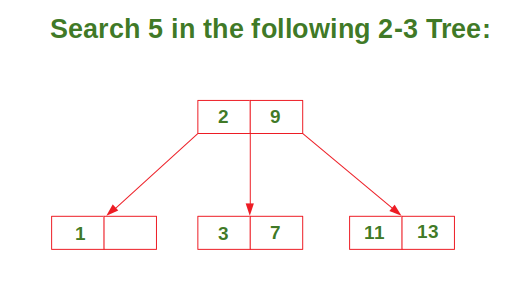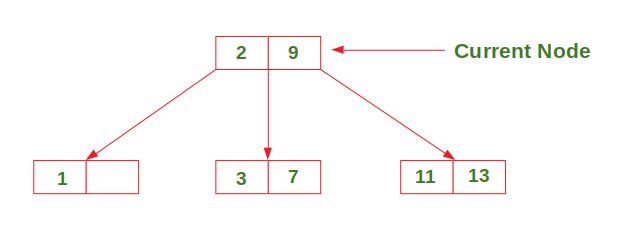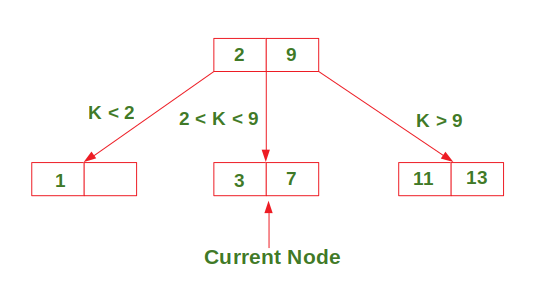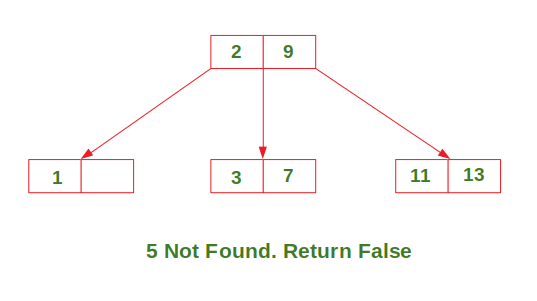Insertion: There are 3 possible cases in insertion which have been discussed below:

Case 1: Insert in a node with only one data element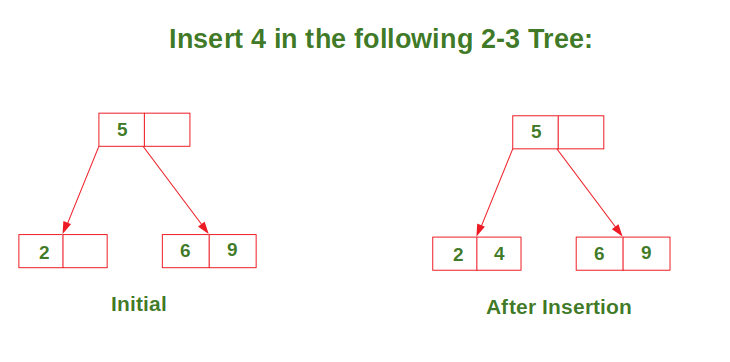Case 2: Insert in a node with two data elements whose parent contains only one data element.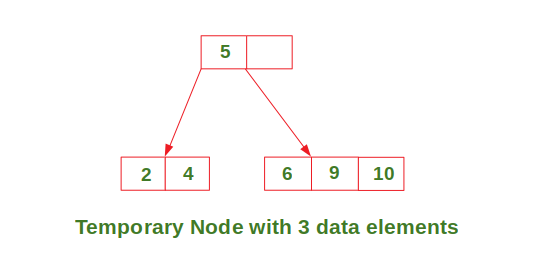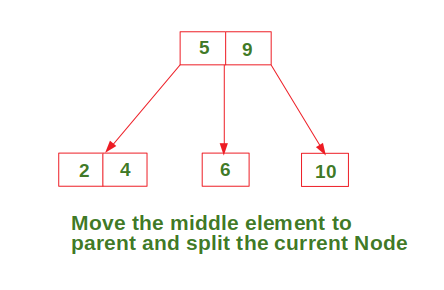Case 3: Insert in a node with two data elements whose parent also contains two data elements.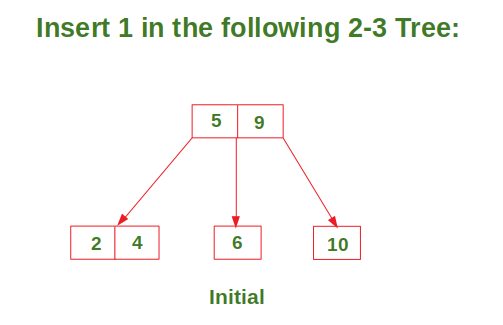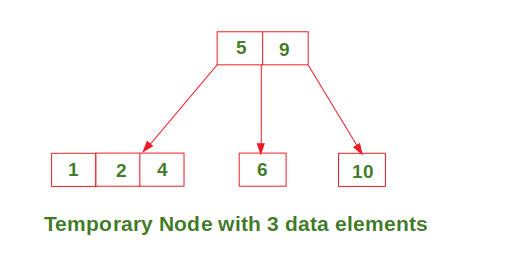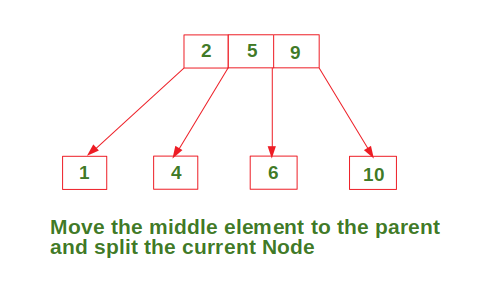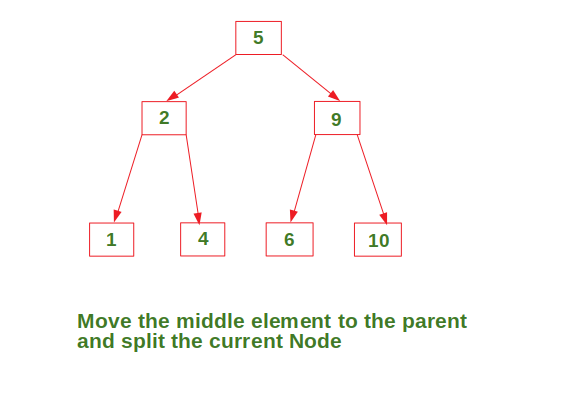My Personal Notes arrow_drop_upCheck out this Author's contributed articles.

If you like GeeksforGeeks and would like to contribute, you can also write an article using contribute.geeksforgeeks.org or mail your article to contribute@geeksforgeeks.org. See your article appearing on the GeeksforGeeks main page and help other Geeks.

Please Improve this article if you find anything incorrect by clicking on the "Improve Article" button below.

Improved By : DuyHoang